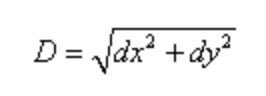# JavaScript Algorithm: Distance Between Points

## Create a function to calculate the distance between two points defined by their x and y coordinates.Photo by Isaac Smith on Unsplash

We are going to write a function called getDistance that will accept four integers (x1, x2, y1, and y2) as arguments.

We are given the coordinates of two points (x1, y1) and (x2, y2). The goal of the function is to return the distance between those two points.

To get the distance of those two points, we use the following formula:dx is the difference between the x-coordinates of the points while dy is the difference between the y-coordinates of the points.

Example:

``````getDistance(100, 100, 400, 300)

// output: 360.5551275463989
``````

In the example above we have point 1: (100,400) and point 2: (100,300)

If we get values of x1 and x2 and subtract the differences, we get dx². If we do the same with y1 and y2 and subtract the differences, we get yx².

Now we can use the formula above to add the differences of x and y together and then square root it to get our answer.

Let’s translate our little pseudocode above into code.

To get our differences between the x-coordinates, we subtract x2 from x1 and assign it to a variable called x.

``````let y = x2 - x1;
``````

We do the same with the y-coordinates:

``````let x = y2 - y1;
``````

Using the formula above, we square x and y. After that, we add their squared results together.

``````x * x + y * y
``````

Next, using the JavaScript method from the Math object, Math.sqrt(), we can square root our result above and return it.

``````return Math.sqrt(x * x + y * y);
``````

Here is the full function:

``````function getDistance(x1, y1, x2, y2) {
let y = x2 - x1;
let x = y2 - y1;

return Math.sqrt(x * x + y * y);
}
``````